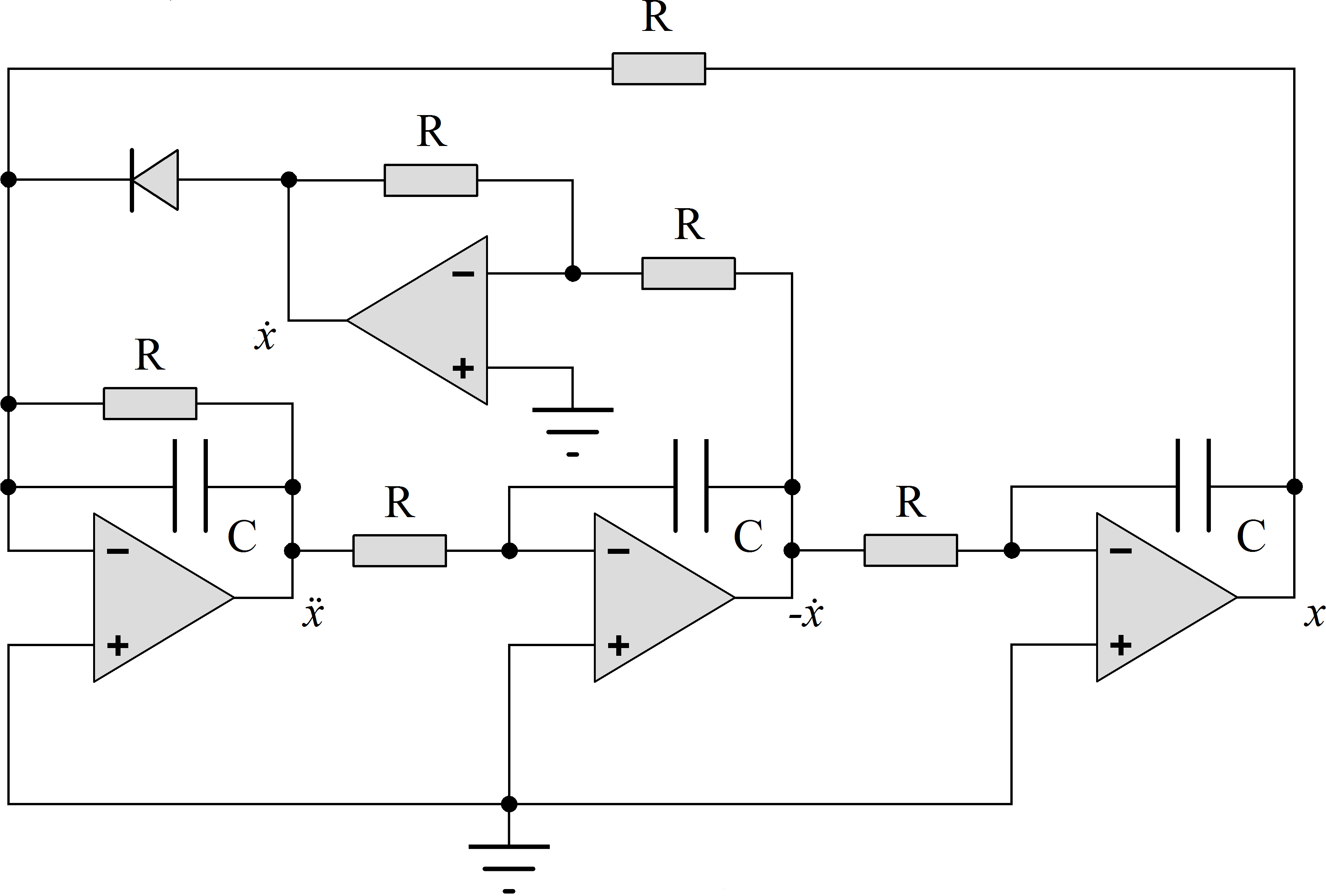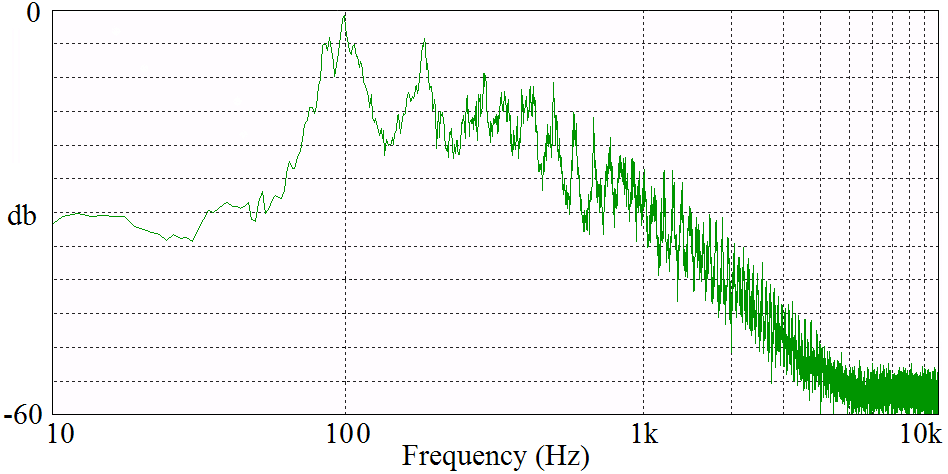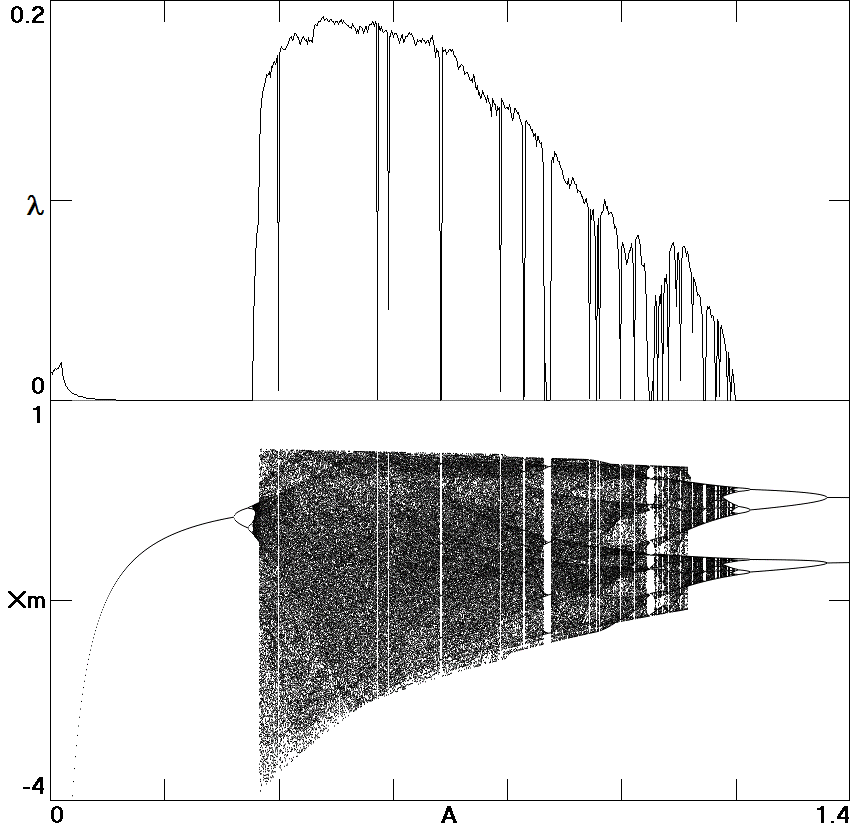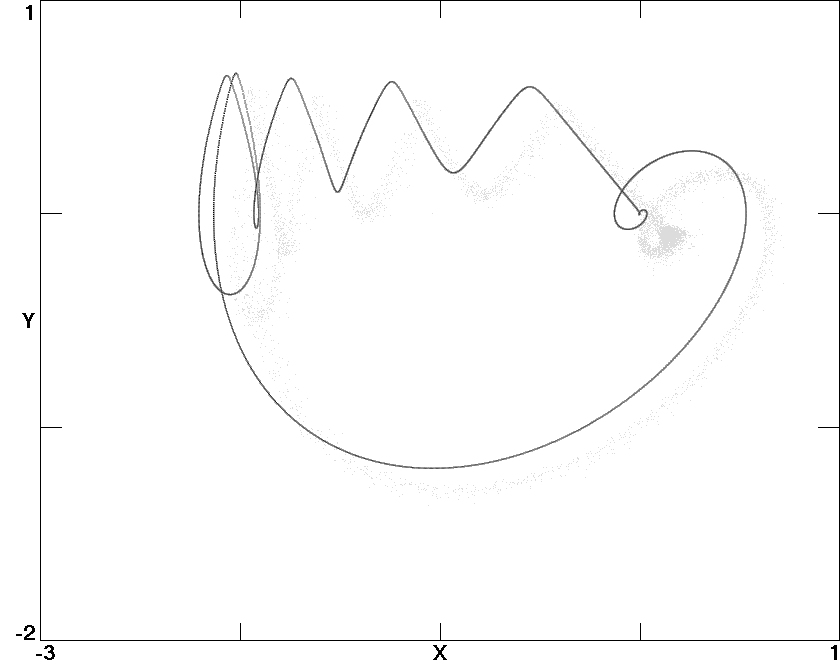# A New Chaotic Jerk Circuit

J. C. Sprott

### ABSTRACT

Much recent interest has been given to simple chaotic oscillators based on jerk equations that involve a third-time derivative of a single scalar variable. The simplest such equation has yet to be electronically implemented. This paper describes a particularly elegant circuit whose operation is accurately described by a simple variant of that equation in which the requisite nonlinearity is provided by a single diode and for which the analysis is particularly straightforward.

(Manuscript received September 20, 2010; revised December 1, 2010; accepted January 26, 2011. Date of current version April 20, 2011.)

Ref: J. C. Sprott, IEEE Transactions on Circuits and Systems--II: Express Briefs 58, 240-243 (2011)

The complete paper is available in PDF format.Fig. 1. Chaotic circuit schematic.Fig. 2. Actual circuit in operation.Fig. 3. Frequency spectrum of the x output of the chaotic circuit.Fig. 4. Numerically calculated phase space plot on the same scale as Fig. 2.Fig. 5. Numerically calculated waveforms of x and its successive derivatives.Fig. 6. Poincaré section in the x-y plane at the instant of maximum diode conduction.Fig. 7. Largest Lyapunov exponent and the value of x when dx/dt is a local maximum versus the bifurcation parameter A in (6).Fig. 8. Homoclinic orbits, with the upper for A = 0.7043 and the lower for A = 0.3890 in (6).